## Rank the following objects by their accelerations down an incline (assume each object rolls without slipping) from least to greatest:

Question

Rank the following objects by their accelerations down an incline (assume each object rolls without slipping) from least to greatest:

a. Hollow Cylinder
b. Solid Cylinder
c. Hollow Sphere
d. Solid Sphere

in progress 0
2 weeks 2021-08-29T19:14:47+00:00 1 Answers 0 views 0

acceleration are

hollow cylinder < hollow sphere < solid cylinder < solid sphere

Explanation:

To answer this question, let’s analyze the problem. Let’s use conservation of energy

Starting point. Highest point

Em₀ = U = m g h

Final point. To get off the ramp

Em_f = K = ½ mv² + ½ I w²

notice that we include the kinetic energy of translation and rotation

energy is conserved

Em₀ = Em_f

mgh = ½ m v² +1/2 I w²

angular and linear velocity are related

v = w r

w = v / r

we substitute

mg h = ½ v² (m + I / r²)

v² = 2 gh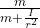v² = 2gh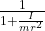this is the velocity at the bottom of the plane ,, indicate that it stops from rest, so we can use the kinematics relationship to find the acceleration in the axis ax (parallel to the plane)

v² = v₀² + 2 a L

where L is the length of the plane

v² = 2 a L

a = v² / 2L

we substitute

a =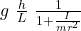let’s use trigonometry

sin θ = h / L

we substitute

a = g sin θ   \ \frac{h}{L} \  \frac{1}{1+ \frac{I}{m r^2 } }

the moment of inertia of each object is tabulated, let’s find the acceleration of each object

a) Hollow cylinder

I = m r²

we look for the acerleracion

a₁ = g sin θ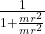1/1 + mr² / mr² =

a₁ = g sin θ    ½

b) solid cylinder

I = ½ m r²

a₂ = g sin θ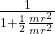= g sin θ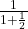a₂ = g sin θ   ⅔

c) hollow sphere

I = 2/3 m r²

a₃ = g sin θ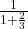a₃ = g sin θd) solid sphere

I = 2/5 m r²

a₄ = g sin θ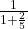a₄ = g sin θWe already have all the accelerations, to facilitate the comparison let’s place the fractions with the same denominator (the greatest common denominator is 210)

a) a₁ = g sin θ ½ = g sin θ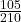b) a₂ = g sinθ ⅔ = g sin θ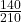c) a₃ = g sin θ= g sin θ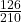d) a₄ = g sin θ= g sin θ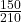the order of acceleration from lower to higher is

a₁ <a₃ <a₂ <a₄

acceleration are

hollow cylinder < hollow sphere < solid cylinder < solid sphere